American Journal of Embedded Systems and Applications
Volume 4, Issue 1, November 2016, Pages: 7-14

Cache Optimization by Fully-Replacement Policy

Chuntao Du1, Xinsong Du2, *

1Department of Computer Science and Technology, North China University of Technology, Beijing, China

2Department of Electrical and Computer Engineering, University of Florida, Gainesville, Florida, USA

Email address: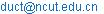(Chuntao Du)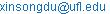(Xinsong Du)

*Corresponding author

To cite this article:

Chuntao Du, Xinsong Du. Cache Optimization by Fully-Replacement Policy. American Journal of Embedded Systems and Applications. Vol. 4, No. 1, 2016, pp. 7-14. doi: 10.11648/j.ajesa.20160401.12

Received: October 20, 2016; Accepted: November 9, 2016; Published: December 5, 2016

Abstract: Cache is an important component in computer architecture. It has great effects on the performance of systems. Nowadays, Least Recently Used (LRU) Algorithm is one of the most commonly used one because it is easy to implement and with a relatively good performance. However, in some particular cases, LRU is not a good choice. To provide references for the computer architecture designer, the study proposed a new algorithm named Fully Replacement Policy (FRP) and then analyzed various factors of effects on cache performance, carried out the simulation experiment of cache performance based on SimpleScalar toolset and SPEC2000 benchmark suite. The study compared the effects of Fully Replacement Policy with Least Recently Used (LRU) Algorithm when set size, block size, associativity and replacement methods are changed separately., By experimentally analyzing the results, it was found that FRP outperforms LRU in some particular situations.

Keywords: Cache memory, Replacement, Optimization, SimpleScaler, SPEC2000

Contents

1. Introduction

Cache as shown in Figure 1 is a high speed and small memory, which is used to store running programs and data. Usually, cache of CPU has two levels: L-1 cache and L-2 cache. Some high performance processors have L-3 cache. In light of recent changes in hardware landscape, we believe that in the future, multilevel caches are invariably going to be hybrid caches where 1) all/most levels are physically collocated 2) the levels differ substantially only with respect to performance and not storage density, and 3) all levels are persistent . Normally, the more cache level or the more capacity, the better performance. It’s very necessary to grasp all kinds of effects on architecture. Software simulation should be a good choice because of the inevitable complexity of hardware implementation.

In order to make room for the new entry on a cache miss, the cache may have to evict one of the existing entries. The heuristic that it uses to choose the entry to evict is called the replacement policy. Replacement policy, one of the key factors determining the effectiveness of a cache, becomes even more important with latest technological trends toward highly associative caches. The state-of-the-art processors employ various policies such as Random, Least Recently Used (LRU), First in First out (FIFO), indicating that there is no common wisdom about the best one.

The development of software is much faster than that of hardware. And the improvement of stream buffer can help us develop computer hardware. Stream buffer is a kind of computer hardware which is between cache L1 and cache L2 and it can, to some extent, reduce the miss rate of cache L1. Stream buffers pre-fetch cache lines when a cache miss exists, and places the data in the buffer. So stream buffers can efficiently decrease the cache pollution and reduce most cache misses in cache L1. When cache L1 miss happens, the computer will start to look for data in stream buffer. If it is a hit, the data would be transferred from stream buffer to cache L1 and then stream buffer would prefetch a new data from cache L2. If it is a miss, all the data in stream buffer will be replaced.

This paper mainly describes the cache performance simulation in order to provide reference for architecture designing, especially for high performance architecture. Through the analysis of the experimental results, the study puts forward the relationship between the cache performance and some cache parameters.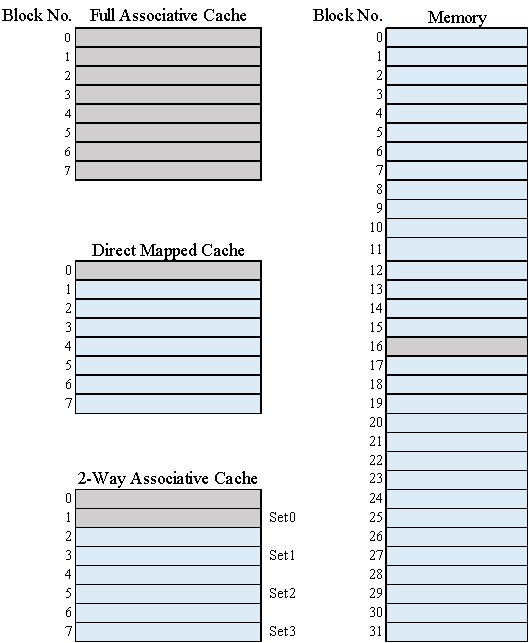Figure 1. Cache and memory.

This paper is organized as follows. Section 2 is related work. Section 3 details the approach. Section 4 is evaluation methodology which contains information about how the study evaluates the above mentioned approach. Section 5 introduces the environmental setups and benchmarks. Section 6 shows the result of experiments and explains "why". Section 7 concludes the report and expands on consideration for future research.

2. Related Work

Nowadays, one of the most common algorithms of cache replacement is LRU. In this algorithm, the frame which has not been used for the longest period of time would be replaced.

Another popular algorithm is Random Replacement Policy, which means that when L1 cache miss happens, the frame that would be replaced is randomly chosen.

Furthermore, First in First out (FIFO) is also commonly used. It replaces frames in cache L1 according to the entered sequence.

To reduce capacity and compulsory misses, Norman P. Jouppi  proposed the idea of stream buffer. With several entries consisting tags, available bits, and data lines, stream buffer implements prefetch before a tag transition take place. However, the study did not implement stream buffer itself. The study tried a new replacement algorithm inspired by the principle of stream buffer.

Waseem Ahmad and Enrico Ng  studied the best processor parameters by SimpleScalar. This research also included the branch prediction, D-cache sizes and the Branch Target Buffers (BTB) preferences. It also proposed some improved methods.

AJ Klein Osowski et al.  compared the behavior of the SPEC 2000 benchmark programs when executing with different input data sets by looking at the fraction of total execution time spent in functions as measured by the profiling tool. They then proposed a method to reduce the execution times of the SPEC 2000 benchmarks in a quantitatively defensible way.

YU Zhi-bin et al.  studied the software simulation technology of architecture. They comprehensively compared and analysed existing simulation technology of architecture in detail.

Much of the compiler tools are simply ports of the GNU software development tools to the SimpleScalar architecture . The ports were written by Todd Austin. The tool set is now supported by Doug Burger, who wrote the documentation for both releases 1.0 and 2.0. Contributions have also been made by Austin B T M et al. , Austin T , and Kalaitzidis K et al .

3. Method

Inspired by stream buffer, the study proposed a new cache replacement algorithm - When a cache miss happens in L1, flush all the data in L1 cache. Due to the spatial locality, if a particular memory location is referenced at a particular time, then it is likely that nearby memory locations will be referenced in the near future. In this case it is common to attempt to guess the size and shape of the area around the current reference for which it is worthwhile to prepare faster access. For example, if data address n missed in L1 cache, it is highly possible that n+1 also missed in this cache. So replace all the data in cache L1 is effective.

In our quest for replacement policy as close to optimal as possible, the study thoroughly explored the design space of existing replacement mechanisms using SimpleScalar toolset and SPEC CPU2000 benchmark suite, across wide range of cache sizes and organizations .

4. Evaluation Methodology

To enhance the performance of cache L1, a new algorithm is proposed by the study. When a miss happens to L1 cache, all the blocks in cache L1 would be replaced. And the study makes the algorithm come true by modifying the code of Simplescalar. This section describes the simulators, our SPEC2000 benchmark suite, and the performance of the new algorithm designed by the study.

4.1. Simulators

To evaluate Fully Replacement Policy, the study employs sim-outorder simulator to generate experimental results. And the study also employs crafty, equake, gzip, swim, vortex and lucas benchmark to test the new algorithm.

4.2. SPEC2000 Benchmark

With the development of CPU, nowadays the study can see that in senior server market different operating system use different CPU. In such a situation, the study employs SPEC CPU 2000 benchmark to test them.

SPEC CPU 2000 is a suite of test programs made by SPEC committee. It can evaluate the performance of CPU by executing a lot of integer and floating point operation in servers. SPEC 2000 consists of two parts: CNIT2000 (Integer test) shown in TABLE 1  and CFP2000 (Floating point test) shown in TABLE 2. 

In our test, the study employs gzip, crafty and vortex from CINT2000. The study also employs equake, lucas and swim from CPF2000 as benchmark.

Table 1. CINT2000 Content.

 Benchmark Language Category 164.gzip C Compression 175.vpr C FPGA Circuit Placement and Routing 176.gcc C C Programming Language Complier 181.mcf C Combinatorial Optimization 186.crafty C Game Playing: Chess 197.parser C Word Processing 252.eon C++ Computer Visualization 253.perlbmk C PERL Programming Language 254.gap C Group Theory, Interpreter 255.vortex C Object-oriented Database 256.bzip2 C Compression 300.twolf C Place and Route Simulator

4.3. Detailed Description of Methodology

To test the effect of our new cache replacement methodology, the study employs contrast test. There are many variables concerning the performance of cache L1. The study in turn changes only one variable while keep others the same. For example, when the study tries to test the influence of block size, the study would only change the value of block size and keep set size, associativity and benchmark unchanged.

Table 2. CFP2000 Content.

 CFP2000 Test Programs Language Description 168.wupwise Fortran 77 Quantum Mechanics 171.swim Fortran 77 Aerography Computation 172.mgrid Fortran 77 Multigrid Solver 173.applu Fortran 77 Parabola/Ellipse Partial Differential Equation 177.mesa C 3-D Graphic Library 178.galgel Fortran 90 Hydromechanics Computation 179.art C Image Recognition/Pivot Network 183.equake C Seismic Wave Simulation 187.facerec Fortran 90 Image Processing: Facial Detection 188.ammp C Chemical Computation 189.lucas Fortran 90 Number Theory 191.fma3d Fortran 90 Collision Simulation 200.sixtrack Fortran 77 High-Energy Atomic Physical Accelerator 301.apsi Fortran 77

4.4. Experiment Procedure

First, set up two folders named simplesim-3.0 and simplesim-3.0R separately. The former one is the original simplescalar-3.0 while the latter one is the modified one. By doing this the study can conveniently compare FRP with LRU.

Secondly, change the code of simplesim-3.0R so that the new algorithm can be implemented. In this simulation, the study edited "cache.c" document and added a new cache replacement algorithm to the system.

Thirdly, open terminal in Ubuntu and compile simplescalar-3.0 and simplescalar-3.0R using config-alpha.

Fourthly, download SPEC2000 Benchmark and place them in the same directory as simplesim-3.0.

Fifth, keep only one variable changed each time and compare the result of FRP with LRU.

Then, make some figures according to the results.

Finally, draw a conclusion.

5. Experiment Setup

The L1 cache of the old system uses LRU to do the replacement while the new system employs FRP. The study employed gzip, crafty and vortex from CINT2000. Equake, lucas and swim from CPF2000 are used as benchmark as well. Then, The effect of FRA in different conditions is able to be tested.

6. Results and Analysis

The study tests miss rates in different benchmarks and compares miss rates in FRP and LRU. These six benchmarks can be divided into two groups: the floating point benchmark (including equake, swim and lucas) and the integer benchmark (including crafty, gzip and vortex). Equake is an application of seismic wave propagation simulation; swim is a shallow water modeling; lucas is an application for number theory or primality testing; crafty is an application for chess game playing; gzip is compression and vortex is an object-oriented database.

Firstly, the study does simulation on the floating point benchmark.

For equake benchmark, when L1 cache block size remains 32 bytes associativity remains 2-way mapping and set size increased gradually, the miss rate of L1 cache is tested. As shown in Figure 2 A, for 64 bytes set size, miss rate of LRU is 2.65% while that of FRP is 2.34, which is 0.31% lower. For 128 bytes set size, the miss rate of LRU is 1.21% while that of FRP is 1.28%, which is 0.07% higher. For the set size 256, the miss rate of LRU is 0.33% while that of FRP is 0.31%, which is 0.02% lower. For 512 bytes set size, the miss rate of LRU is 0.27% while that of FRP is 0.26, which is 0.01% lower. For 1024 bytes set size of, the miss rate of LRU is 0.25% while that of FRP is also 0.25%.

For equake benchmark, when L1 cache set size remains 64 bytes, associativity remains 2-way mapping and block size increases gradually, the miss rate of L1 cache is tested. As shown in figure 2 B, for 8 bytes block size, the miss rate of LRU is 13.04% while that of FRP is 13.87%, which is 0.83% higher. For 16 bytes block size, the miss rate of LRU is 5.15% while that of FRP is 5.45%, which is 0.30% higher. For 32 bytes block size, the miss rate of LRU is 2.65% while that of FRP is 2.55%, which is 0.10% lower. For 64 bytes block size, the miss rate of LRU is 1.94% while that of FRP is 2.04%, which is 0.10% higher.

For equake benchmark, when the block size remains 32 bytes, set size remains 64 bytes and associativity increases gradually, the miss rate of L1 cache is tested. As shown in Figure 2 C For 2-way mapping, the miss rate of LRU is 2.65% while that of FRP is 2.55%, which is 0.10% lower. For 4-way associativity, the miss rate of LRU is 0.40% while that of FRP is 2.55%, which is 2.15% higher. For 8-way associativity, the miss rate of LRU is 0.29% while that of FRP is 2.55%, which is 1.96% higher. For 16-way associativity, the miss rate of LRU is 0.26% while that of FRP is 2.55%.

For lucas benchmark, when the block size remains 32 bytes, associativity remains 2-way mapping and set size increases gradually, the miss rate of L1 cache is tested. As shown in Figure 2 D, for 64 bytes set size, the miss rate of LRU is 2.63% while that of FRP is 2.66%, which is 0.03% higher. For 128 bytes set size, the miss rate of LRU is 1.25% while that of FRP is 1.18%, which is 0.07% lower. For the set size of 256, the miss rate of LRU is 0.94% while that of FRP is 0.91%, which is 0.03% lower. For 512 bytes set size, the miss rate of LRU is 0.83% while that of FRP is 0.81%, which is 0.02% lower. For 1024 bytes set size, the miss rate of LRU is 0.77% while that of FRP is 0.76%, which is 0.01% lower.

For the lucas benchmark, when the set size remains 64 bytes, associativity remains 2-way mapping and block size increases gradually, the miss rate of L1 cache is tested. As shown in Figure 2 E, for 8 bytes block size, the miss rate of LRU is 6.89% while that of FRP is 6.90%, which is 0.01% higher. For 16 bytes block size, the miss rate of LRU is 4.94% while that of FRP is 5.00%, which is 0.06% higher. For 32 bytes block size, the miss rate of LRU is 2.63% while that of FRP is 2.66%, which is 0.03% higher. For 64 bytes block size, the miss rate of LRU is 0.91% while that of FRP is 0.87%, which is 0.04% lower.

For the lucas benchmark, when the set size remains 64 bytes, block size remains 32 bytes and associativity increases gradually, the miss rate of L1 cache is tested. For 2-way mapping, the miss rate of LRU is 2.63% while that of FRP is 2.66%, which is 0.03% higher. As shown in Figure 2 F, for 4-way mapping, the miss rate of LRU is 1.31% while that of FRP is 2.66%, which is 1.35% higher. For 8-way mapping, the miss rate of LRU is 0.95% while that of FRP is 2.66%, which is 1.71% higher. For 16-way mapping, the miss rate of LRU is 0.82% while that of FRP is 2.66%, which is 1.84% higher.

For the swim benchmark, when the set size remains 32 bytes, associativity remains 2-way mapping and block size increases gradually, the miss rate is tested. As shown in Figure 2 G, for 64 bytes block size bytes, the miss rate of LRU is 1.74% while that of FRP is 1.66%, which is 0.08% lower. For the block size of 128 bytes, the miss rate of LRU is 1.38% while that of FRP is 1.34%, which is 0.04% lower. For the block size of 256, the miss rate of LRU is 1.22% while that of FRP is 1.20%, which is 0.02% lower. For the 512 bytes block size, the miss rate of LRU is 1.14% while that of FRP is 1.13%. For 1024 bytes block size, the miss rate of LRU is 1.10% while that of FRP is 1.09%, which is 0.01% lower.

For swim benchmark, when set size remain 64 bytes, associativity remains 2-way mapping and block size increased gradually, the miss rate is tested. As shown in Figure 2 H, for 8 bytes block size, the miss rate of LRU is 11.60% while that of FRP is 11.94%, which is 0.34% higher. For 16 bytes block size, the miss rate of LRU is 5.55% while that of FRP is 5.54%. For 32 bytes block size, the miss rate of LRU is 1.74% while that of FRP is 1.66%, which is 0.08 lower. For 64 bytes block size, the miss rate of LRU is 1.28% while that of FRP is 1.33%, which is 0.05% higher.

For swim benchmark, when set size remains 64 bytes, block size remains 32 bytes and associativity increases gradually, the miss rate is tested. As shown in Figure 2 I, when the associativity is 2-way mapping, the miss rate of LRU is 1.74% while that of FRP is 1.66%, which is 0.08% lower. When the associativity is 4-way mapping, the miss rate of LRU would be 1.34% while that of FRP would be 1.66%, which is 0.32% higher. When the associativity is 8-way mapping, the miss rate of LRU would be 1.19% while that of FRP is 1.66%, which is 0.47%.

Secondly, the study does simulation on the integer benchmark.

For crafty benchmark, when block size remains 32 bytes, associativity remains 2-way mapping and set size increases gradually, the miss rate is tested. As shown in Figure 2 J, when the set size is 64 bytes, the miss rate of LRU is 14.82% while that of FRP is also 14.82%. When the set size is 128 bytes, the miss rate of LRU is 9.11% while that of FRP is 9.13%, which is 0.02% higher. When the set size is 256 bytes, the miss rate of LRU is 4.49% while that of FRP is 4.50%, which is 0.01% higher. When the set size is 512 bytes, the miss rate of LRU is 2.33% while that of FRP is 2.29%, which is 0.04% lower. For 1024 bytes set size, the miss rate of LRU is 1.30% while that of FRP is 1.25%, which is 0.05% lower.

For crafty benchmark, when set size remains 64 bytes, associativity remains 2-way mapping and block size increases gradually, the miss rate is tested. As shown in Figure 2 K, for 8 bytes block size, the miss rate of LRU is 32.84% while that of FRP is 32.74%, which is 0.10% lower. For 16 bytes block size, the miss rate of LRU is 21.70% while that of FRP is 21.94%, which is 0.24% higher. For 32 bytes block size, the miss rate of LRU is 14.82% while that of FRP is also 14.82%. For 64 bytes block size, the miss rate of LRU is 11.05% while that of FRP is 11.09%, which is 0.04% higher.

For crafty benchmark, when set size remains 64 bytes, block size remains 32 bytes and associativity increases gradually, the miss rate is tested. As shown in Figure 2 L, for 2-way mapping, the miss rate of LRU is 14.82% while that of FRP is also 14.82%. For 4-way mapping, the miss rate of LRU is 7.20% while that of FRP is 14.82%, which is 7.62% higher. For 8-way mapping, the miss rate of LRU is 2.85% while that of FRP is 14.82%, which is 11.97% higher. For 16-way mapping, the miss rate of LRU is 1.25% while that of FRP is 14.85%, which is 13.60% higher.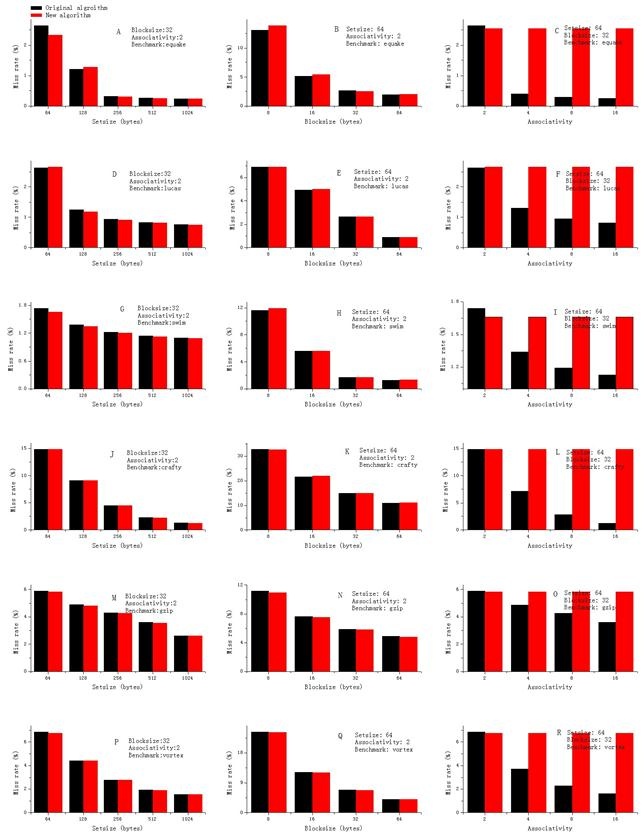Figure 2. L1 cache miss rate of LRU and FRP in different conditions.

For gzip benchmark, when block size remains 32 bytes, associativity remains 2-way mapping, set size increases gradually, the miss rate is tested. As shown in Figure 2 M, for 64 bytes set size, the miss rate of LRU is 5.90% while that of FRP is 5.83%, which is 0.07% lower. For 128 set size, the miss rate of LRU is 4.89% while that of FRP is 4.81%, which is 0.08% lower. For 256 set size, the miss rate of LRU is 4.29% while that of FRP is 4.24%, which is 0.05% lower. For 512 bytes block size, the miss rate of LRU is 3.59% while that of FRP is 3.56%, which is 0.03% lower. For 1024 bytes block size, the miss rate of LRU is 2.63% while that of FRP is 2.60%, which is 0.03% lower.

For gzip benchmark, when set size remains 64 bytes, associativity remains 2-way mapping and block size increases gradually, the miss rate is tested. As shown in Figure 2 N, for 8 bytes block size, the miss rate of LRU is 11.22% while that of FRP is 10.99%, which is 0.23% lower. For 16 bytes block size, the miss rate of LRU is 7.67% while that of FRP is 7.53%, which is 0.14% lower. For 32 bytes block size, the miss rate of LRU is 5.9% while that of FRP is 5.83%, which is 0.07% lower. For 64 bytes block size, the miss rate of LRU is 4.88% while that of FRP is 4.80%, which is 0.08% lower.

For gzip benchmark, when block size remains 32 bytes, set size remains 64 bytes and associativity increases gradually, the miss rate is tested. As shown in Figure 2 O, for 2-way mapping, the miss rate of LRU is 5.90% while that of FRP is 5.83%, which is 0.07% lower. For 4-way mapping, the miss rate of LRU is 4.85% while that of FRP is 5.83%, which is 0.98% higher. For 8-way mapping, the miss rate of LRU is 4.26% while that of FRP is 5.83%, which is 1.57% higher. For 16-way mapping, the miss rate of LRU is 3.6% while that of FRP is 5.83%, which is 2.23% higher.

For vortex benchmark, when block size remains 32 bytes, associativity remains 2-way mapping and set size increases gradually, the miss rate is tested. As shown in Figure 2 P, for 64 bytes set size, the miss rate of LRU is 6.86% while that of FRP is 6.76%, which is 0.10% lower. For 128 bytes set size, the miss rate of LRU is 4.43% while that of FRP is 4.41%, which is 0.02% lower. For 256 bytes set size, the miss rate of LRU is 2.79% while that of FRP is 2.76%, which is 0.03% lower. For 512 bytes set size, the miss rate of LRU is 1.94% while that of FRP is 1.92%, which is 0.02% lower. For 1024 bytes set size, the miss rate of LRU is 1.54% while that of FRP is 1.53%, which is 0.01% lower.

For vortex benchmark, when set size remains 64 bytes, associativity remains 2-way mapping and block size increases gradually, the miss rate is tested. For 8 bytes block size, the miss rate of LRU is 24.33% while that of FRP is 24.20%, which is 0.13% lower. As shown in Figure 2 Q, for 16 bytes block size, the miss rate of LRU is 12.21% while that of FRP is 12.05%, which is 0.16% lower. For 32 bytes block size, the miss rate of LRU is 6.86% while that of FRP is 6.76%, which is 0.10% lower. For 64 bytes block size, the miss rate of LRU is 4.00% while that of FRP is 3.97%, which is 0.03% lower.

For vortex benchmark, when set size remains 64 bytes, block size remains 32 bytes and associativity increases gradually, the miss rate is tested. As shown in Figure 2 R, for 2-way mapping, the miss rate of LRU is 6.86% while that of FRP is 6.76%, which is 0.10% lower. For 4-way mapping, the miss rate of LRU is 3.71% while that of FRP is 6.76%, which is 3.05% higher. For 8-way mapping, the miss rate of LRU is 2.29% while that of FRP is 6.76%, which is 4.47% higher. For 16-way mapping, the miss rate of LRU is 1.62% while that of FRP is 6.76%, which is 5.14% higher.

Based on the above benchmark tests, miss rate are shown in these figures when L1 set size is changed from 64 to 1024, the L1 block size is changed from 8 bytes to 64 bytes and the L1 associativity is changed from 2-way to 16-way. The selected results and relative parameters are shown as Figure 1-Figure4.Figure 3. L1 Cache miss rate improvement when using FRP comparing to LRU.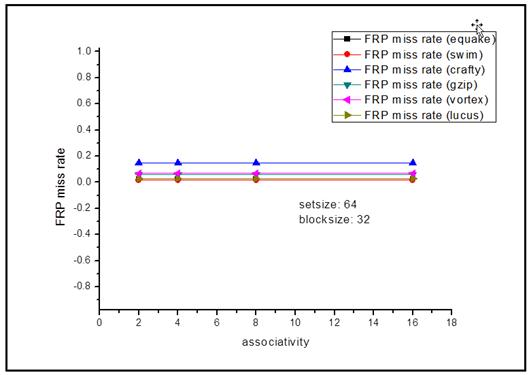Figure 4. The relationship between associativity and L1 cache miss rate when using FRP.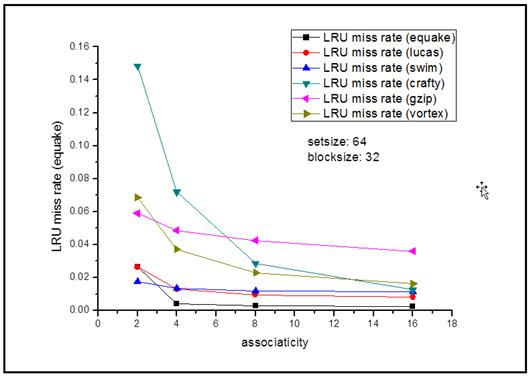Figure 5. The relationship between associativity and L1 cache miss rate when using LRU.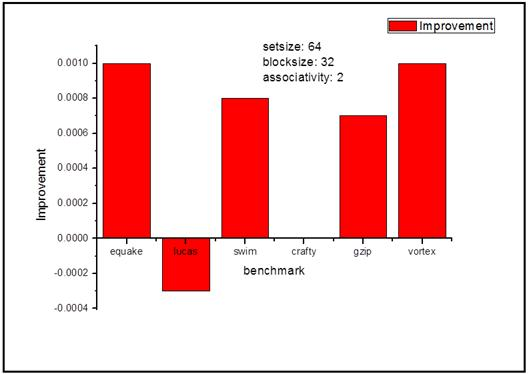Figure 6. The improvement of L1 cache miss rate when using FRP in the condition of 64 bytes set size, 32 bytes block size and 2-way mapping associativity.

7. Conclusion

According to Figure 1, it is obviously that when the L1 cache has a block size of 32 byte and with a 2-way associativity, Fully Replacement Policy works better than LRU most of the time, especially when the setsize is small. Besides, when using Fully Replacement Policy, miss rate has nothing to do with the associativity of L1 cache while miss rate decreases as the increase of associativity when using LRU, which means that the less the associativity is, the better to use Fully Replacement Policy than LRU. According to Figure 4, FRP works better than LRU for most benchmarks when the setsize is 64, blocksize is 32 and associativity is 2.

Acknowledgements

This research is supported by the key projects of education and teaching reform in North China University of Technology in 2016 (Grant Number: XN093-002), Google supports the Industry-University Cooperation professional comprehensive reform project of the Department of higher education of the Ministry of Education in 2016, Project of the 2016 National Institute of computer basic education (Grant Number: 2016042), and the subproject of Microsoft supports the Industry-University Cooperation professional comprehensive reform project of the Department of higher education of the Ministry of Education in 2015.

References

1. Appuswamy R, Moolenbroek D C V, Tanenbaum A S. Cache, cache everywhere, flushing all hits down the sink: On exclusivity in multilevel, hybrid caches[J]. 2013:1-14.
2. Jouppi, Norman P."Improving Direct-Mapped Cache Performance by the Addition of a SmallFully-Associative Cache and Prefetch Buffers." In Computer Architecture,1990. Proceedings., 17th Annual International Symposium on, 364–73. IEEE,1990.Geoffrey E. Hinton, and Ruslan R. Salakhutdinov. Reducing the dimensionality of data with neural networks. Science 313.5786 (2006): 504~507.
3. Waseem Ahmad, Enrico Ng. "A Quantitative/Qualitative Study for Optimal Parameter Selection of a Superscalar Processor using SimpleScalar". Computer Sciences Technical Report, 2004.
4. A.J Klein Osowski, J. Flynn, N. Meares, and D.J. Lilja. Adapting the SPEC2000 benchmark suite for simulation-based computer architecture research. In Workshop on Workload Characterization, 2000.
5. Yu Zhi-Bin, Jin Hai, Zou Nan-Hai. "Computer architecture software-based simulation". Journal of Software, 2008,19(4), pp. 1051-1068.
6. Doug Burger, Todd M. Austin, The SimpleScalar tool set, version 2.0, ACM SIGARCH Computer Architecture News, v.25 n.3, p.13-25, June 1997 [doi>10.1145/268806.268810].
7. Austin B T M, Burger D, Franklin M, et al. Skadron, "The SimpleScalar Architectural Research Tool Set," http://www.cs.wisc.edu/~mscalar/ simplescalar.html,retrieved April 24[J]. 2010.
8. Austin T, Larson E, Dan E. Simplescalar: "An Infrastructure for Computer System Modeling"[J]. Computer, 2002, 35(2):59-67.
9. Kalaitzidis K, Dimitriou G, Stamoulis G, et al. Performance and power simulation of a functional-unit-network processor with simplescalar and wattch[C]// The, Panhellenic Conference. 2015:71-76.
10. J. Cantin, and M. Hill, "Cache Performance for SPEC CPU 2000 Benchmarks"http://www.cs.wisc.edu/multifacet/miso/spec2000 cache-data/
11. S. Sair and M. Charney. Memory behavior of the SPEC2000 benchmark suite. Technical report, IBM, 2000.
12. D. Citron, "MisSPECulation: Partial and Misleading Use of SPEC CPU2000 in Computer Architecture Conferences", Proc. of International Symposium on Computer Architecture, pp. 52-61, 2003.

 Contents 1. 2. 3. 4. 4.1. 4.2. 4.3. 4.4. 5. 6. 7.
Article ToolsAbstractPDF(473K)
Follow on us
PUBLICATION SERVICE
JOIN US
RESOURCES
SPECIAL SERVICES
ADDRESS
Science Publishing Group
548 FASHION AVENUE
NEW YORK, NY 10018
U.S.A.
Tel: (001)347-688-8931